Courses

# RD Sharma Solutions -Ex-11.2, Angles, Class 6, Maths Class 6 Notes | EduRev

## RD Sharma Solutions for Class 6 Mathematics

Created by: Abhishek Kapoor

## Class 6 : RD Sharma Solutions -Ex-11.2, Angles, Class 6, Maths Class 6 Notes | EduRev

The document RD Sharma Solutions -Ex-11.2, Angles, Class 6, Maths Class 6 Notes | EduRev is a part of the Class 6 Course RD Sharma Solutions for Class 6 Mathematics.
All you need of Class 6 at this link: Class 6

Q.1) Give two examples each of right, acute and obtuse angles from your environment.

Sol.1 : Two examples of right angle in our environment are:

(i) The angle formed by the two adjacent walls of a room is a right angle.

(ii) The angle formed by the two adjacent edges of a book is a right angle.

Two examples of acute angle in our environment are:

(i) The angle formed between the two adjacent fingers of our hand.

(ii) The angle between the two adjacent sides of the letter Z of English alphabet.

Two examples of obtuse angle in our environment are:

(i) The smaller angle formed by the two adjacent blades of a fan.

(ii) The smaller angle formed by the two sloping sides of a roof of a but is an obtuse angle.

Q.2 An angle is formed by two adjacent fingers. What kind of angle will it appear?

Sol.2 : Angle formed by two adjacent fingers will appear as an acute angle.

Q.3 Shikha is rowing a boat due northeast. In which direction will she be rowing if she turns it through : (i) a straight angle. (ii) a complete angle.

Sol.3 : (i) If Shikha turns through a straight angle or 180 degrees, she will be rowing along the south – west direction.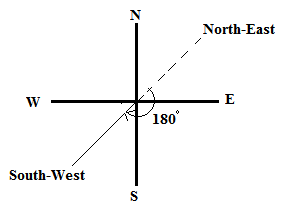(ii) If Shikha turns through a complete angle or 360 degrees, she will be rowing along her original direction, i.e., north – east direction.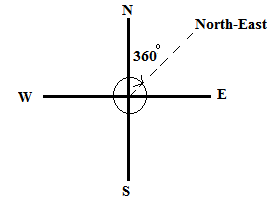Q.4 What is the measure of the angle in degrees between :

(i) North and West?

(ii) North and South?

(iii) North and South – East?

Sol.4 : The measure of the angle between :

(i) North and West is 90 degrees.

(ii) North and South is 180 degrees.

(iii) North and South – East is 135 degrees.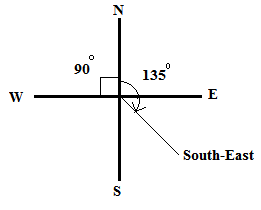Q.5 A ship sailing in river Jhelam moves towards east. If it changes to north, through what angle does it turn?

Sol.5 : If the ship is sailing in east direction and turns to north direction, it turns through an angle of 90 degrees.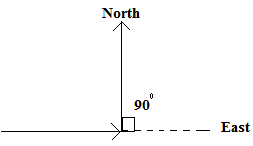Q.6 You are standing in a class room facing north. In what direction are you facing after making a quarter turn?

Sol.6 : After making a quarter turn or a turn of 90 degrees, i will be facing east if i turn to my right hand. Similarly, if i turn to my left hand, i will be facing west.

Q.7 A bicycle wheel makes four and a half turns. Find the number of right angles through which it turns.

Sol.7 : In one turn, the wheel of a bicycle covers 360°.

If we express 360° in right angles, we get :

360°/90° = 4 right angles.

Thus, in four and a half turns, the wheel will turn by (4 x 4.5) = 18 right angles.

Q.8 Look at your watch face. Through how many right angles does the minute hand moves between 8 O’ clock and 10:30 O’ clock?

Sol.8 : The time interval between 8 : 00 O'clock and 10 : 30 O'clock is 2.5 hours, i.e., two and a half hours.

In 1 hour, the minute hand turns by a complete angle, i.e., 360° or 360°/90° = 4 right angles.

Thus, in 2.5 hours, the minute hand will turn by 2.5 x 4 = 10 right angles.

Q.9 If a bicycle wheel has 48 spokes, then find the angle between a pair of adjacent spokes.

Sol.9 : In a bicycle, the central angle measures 3600 and it consists of 48 spokes.

Therefore, angle between any two adjacent spokes = 360/48 = 7.50.

Q.10 Classify the following angles as acute, obtuse, straight, right, zero and complete angle:

(i) 1180

(ii) 290

(iii) 1450

(iv) 1650

(v) 00

(vi) 750

(vii) 1800

(viii) 89.50

(ix) 300

(x) 900

(xi) 1790

(xii) 3600

(xiii) 90.50

Sol.10 : An acute angle measures between 0° and 90° ; an obtuse angle measures between 90° and 180° ; a straight angle measures 180° ; a right angle measures 90° ; a zero angle measures 0° and a complete angle measures 360°.

(i) 118° is an obtuse angle.

(ii) 29° is an acute angle.

(iii) 145° is an obtuse angle.

(iv) 165° is an obtuse angle.

(v) 0° is a zero angle.

(vi) 75° is an acute angle.

(vii) 180° is a straight angle.

(viii) 89.5° is an acute angle.

(ix) 30° is an acute angle.

(x) 90° is a right angle.

(xi) 179° is an obtuse angle.

(xii) 360° is a complete angle.

(xiii) 90.5° is an obtuse angle.

Q.11 Using only a ruler, draw an acute angle, a right angle and an obtuse angle in your notebook and name them.

Sol.11 :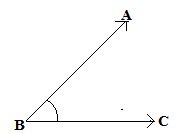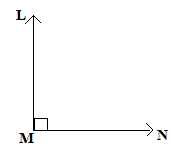Right angle ∠LMN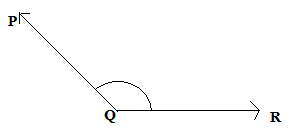Obtuse angle ∠PQR

Q.12 State the kind of angle, in each case, formed between the following directions :

(i) East and West

(ii) East and North

(iii) North and North - East

(iv) North and South - East

Sol.12: (i) East and west directions form an angle of 180°, which is a straight angle.

(ii) East and north directions form an angle of 90°, which is a right angle.

(iii) North and north-east directions form an angle of 45°, which is an acute angle.

(iv) North and south-east directions form an angle of 135°, which is an obtuse angle.

Q.13 State the kind of each of the following angles :

Sol.13 :

(i) Acute angle, as it measures between 0° and 90°.

(ii) Obtuse angle, as it measures between 90° and 180°.

(iii) Straight angle, as it is equal to 180°.

(iv) Right angle, as it is equal to 90°.

(v) Complete angle, as it is equal to 360°.

OBJECTIVE TYPE QUESTIONS :

Mark the correct alternative in each of the following :

Q.1. The vertex of an angle lies

(a) in its interior (b) in its exterior (c) on the angle (d) inside the angle

Sol.1 : (c) on the angle.

The vertex of an angle lies on the angle.

Q.2. The figure formed by two rays with the same initial point is known as

(a) a ray (b) a line (c) an angle (d) a line segment

Sol.2 : (c) an angle.

An angle is a figure by two rays with the same initial point.

Q.3. An angle of measure 00 is called

(a) a complete angle (b) a right angle (c) a straight angle (d) none of these

Sol.3 : (d) none of these.

An angle of measure 00 is called a zero angle.

Q.4. An angle of measure 900 is called

(a) a complete angle (b) a right angle (c) a straight angle (d) a reflex angle

Sol.4 : (b) a right angle.

An angle of measure 900 is called a right angle.

Q.5. An angle of measure 1800 is called

(a) a zero angle (b) a right angle (c) a straight angle (d) a reflex angle

Sol.5 : (c) a straight angle.

An angle of measure 1800 is a straight angle.

Q.6. An angle of measure 3600 is called

(a) a zero angle (b) an straight angle (c) a reflex angle (d) a complete angle

Sol.6 : (d) a complete angle.

An angle of measure 3600 is called a complete angle.

Q.7. An angle of measure 2400 is

(a) an acute angle (b) an obtuse angle (c) a straight angle (d) a complete angle

Sol.7 : None of the given options are correct.

An angle of measure 2400 is called a reflex angle.

Q.8. A reflex angle measures

(a) more than 900 but less than 1800 (b) more than 1800 but less than 2700 (c) more than 1800 but less than 360(d) none of these.

Sol.8 : (c) more than 1800 but less than 3600

A reflex angle is defined as an angle that measures more than 1800 but less than 3600.

Q.9. The number of degrees in 2 right angles is

(a) 90(b) 1800 (c) 2700 (d) 3600

Sol.9 : (b) 1800

Since, 1 right angle = 900

Therefore, 2 right angles = 900 x 2 = 1800

Q.10. The number of degrees in 3/2 right angles is

(a) 180(b) 360(c) 2700 (d) 900

Sol.10 : None of the options are correct.

Since, 1 right angle = 900

Therefore, 3/2 right angles = 3/2 x 90= 1350

Q.11. If bicycle wheel has 36 spokes, then the angle between a pair of adjacent spokes is

(a) 100 (b) 150 (c) 200 (d) 120

Sol.11 : (a) 100

The complete angle of bicycle wheel measures is 3600.

Therefore, the angle between two adjacent spokes of the containing 36 spokes = 360/36 = 100.

103 docs

,

,

,

,

,

,

,

,

,

,

,

,

,

,

,

,

,

,

,

,

,

,

,

,

,

,

,

,

,

,

;